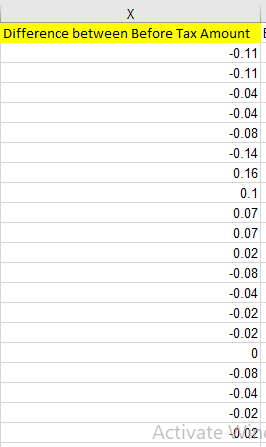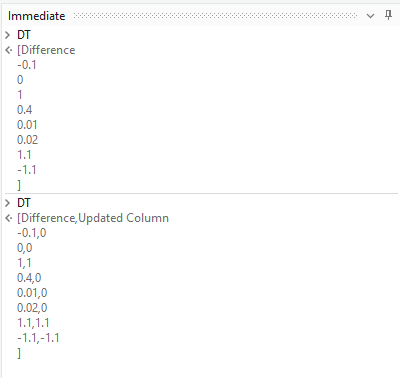# Update a column in a datatable using LINQ

Hi Team,Above is my column X, i have a condition that if the value of this column is greater than -1 and less than 1 , then i will replace the current value with 0 otherwise leaving the value as it is.

@supermanPunch any help?

@yash.choursia

updatedDataTable = (From row In dataTable.AsEnumerable()
Let value = Convert.ToDouble(row(“Column_X”))
Let updatedValue = If(value > -1 AndAlso value < 1, 0, value)

write the new dt based on condition using below linq

syntax : DT.AsEnumerable.Where(Function(r) if(double.parse(r(column name).ToString) < 1 andalso double.parse(r(columnname).ToString) > -1 ,True,False)).CopyToDataTable

and write the new dt values as zero using below linq

syntax : New DT.AsEnumerable.select(Function(r) New DT.Clone.LoadDataRow(New Object(){r(0).ToString,“0”},false)).copytodatatable

``````Assign activity:
updatedColumnX = columnX.Select(Function(x) If(Double.TryParse(x.ToString(), outValue),
If(outValue >= -1 AndAlso outValue <= 1, 0, outValue),
x)).ToArray()

``````

Use invoke code activity

For Each r As DataRow In in_dt.AsEnumerable
r(“Difference between Before Tax Amount”)=If((Math.Abs(CDbl(r(“Difference between Before Tax Amount”).ToString)))<1, “0”, r(“Difference between Before Tax Amount”).ToString)
Next r

Cross Reference:

An Alternate with Data Column Expression (As it is an easier/simpler operation) :

Implementation :

Here, Notice the use of `Add Data Column` to add another column for updating the newer values.

We then use the Data Column Expression using Assign in the below Way :

``````DT.Columns("Updated Column").Expression = "IIF(CONVERT([Difference],'System.Double')>-1 And CONVERT([Difference],'System.Double')<1,0,CONVERT([Difference],'System.Double'))"
``````

We can also simply remove the Older Column with `DefaultView.ToTable()` and rename the new column to the old one. (We do see additional steps involved)

Debug Panel :This topic was automatically closed 3 days after the last reply. New replies are no longer allowed.